Applied Statistics: Descriptive Statistics II

Having studied different ways of representing the statistical mean of a data set, we now turn to other aspects of characterizing data. Specifically, we consider variance and standard deviation as measures of dispersion and skewness as a measure of asymmetry. We also consider moments about the mean as a general tool used in calculating these statistical values.

Key Terms

o         Moment about the mean (or moment)

o         Measure of dispersion

o         Variance

o         Standard deviation

o         Estimator

o         Skewness

o         Measure of asymmetry

Objectives

o         Use moments about the mean to represent measures of dispersion and asymmetry

o         Calculate the variance, standard deviation, and skewness of data sets

Let's Begin!

The central tendency (such as the arithmetic or geometric mean) of a data set is a helpful tool, but it is unable to distinguish characteristics beyond the location of the "middle" of the data. For instance, the two data sets below have the same arithmetic mean, but the data are distributed in drastically different ways around that mean:

{1.01, 1.01, 1.03, 1.05, 1.07, 1.09, 1.11, 1.13, 1.13}

{0.01, 0.02, 0.05, 0.16, 1.07, 1.98, 2.09, 2.12, 2.13}

In both cases, the arithmetic mean is 1.07 (the middle value in the ordered sets), but the data distributions around that mean are different. For the first set, the data are all very close to the mean (within 0.06), whereas for the second set, the data are mostly distant from the mean (at least 0.91). Thus, to better describe data sets, we must also find a way to numerically describe (using a single value) how data are spread around the mean--whether it is generally close to the mean (as in the first case) or generally far from the mean (as in the second case).

In addition, note that the data in both sets are distributed symmetrically about the mean. This is not always the case, however, so we also want to be able to numerically quantify the symmetry of a data set. To this end, we will look generally in this article at so-called moments, and specifically, we will consider the variation (spread) and skewness (asymmetry) of a set of data. Later, we will take what we have learned and apply it to frequencies.

A moment about the mean (or simply moment) is defined mathematically as follows for a data set containing N values. The natural number k identifies the particular moment (in other words, the expression below is for the kth moment about the mean)--thus, we use the expression μk to identify this moment.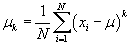In the above expression, xi is once again the ith value in the data set, and μ is the arithmetic mean. For example, the second moment about the mean, μ2, is the following: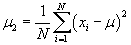Note that when the value of k is specified, we can calculate μk for any given data set. At this point, the concept of a moment is abstract, and its precise relationship to descriptive statistics may be unclear. Let's now consider how we can use the concept of a moment to characterize a data set-specifically, we'll consider the variance.

Practice Problem: What is the expression for the fifth moment about the mean for a data set containing n values?

Solution: Simply use the expression for μk given here-in this case, let k be 5, and also substitute n for N. In the remainder of the article, we will use moments to statistically describe data sets.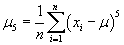Interested in learning more? Why not take an online Applied Statistics course?

Variance and Standard Deviation

We already know how to find the "middle" of a data set. Our next task is to describe how those data are spread (or "dispersed") about the middle-such a value is called a measure of dispersion. We can first consider the "distance" of each data value xi from the mean μ--this distance is simply the difference xi – μ. One possible measure of dispersion would be the arithmetic mean of the distances of all the data values from the mean of the data set: we would simply calculate all the distances xi – μ, sum them, and then divide by N (the total number of values). The corresponding mathematical expression is shown below.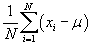This expression is an average distance of the data values from the mean, but it has one problem: if the data values are distributed symmetrically about the mean (as they are in the example sets at the beginning of the article), then this value becomes zero. Let's illustrate using the first example data set from above: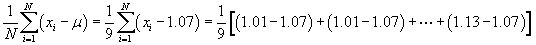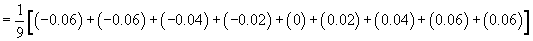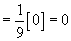But a value of zero is not helpful; hence, we use the square of the distance to eliminate the signs. Thus, a more useful formula is the following--this value is called the variance, and it is expressed as σ2.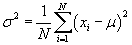Specifically, this is the population variance, since we are assuming that the data set corresponds to a population rather than a sample of the population. Here, we also see the practical relationship between moments and variance--the variance of a data set is the same as the second moment about the mean of that set. Later in the article, we will also use moments to quantify the symmetry (or asymmetry) of a data set.

The square root of the variance expression above is the standard deviation; this value corresponds more to an average distance rather than an average square distance, although this analogy is not perfectly accurate. We represent the population standard deviation as σ.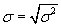Practice Problem: Calculate the standard deviation of the second example data set from the beginning of the article (assume that the data set is a population).

Solution: First, we must calculate the variance; the square root of this result is the standard deviation.

{0.01, 0.02, 0.05, 0.16, 1.07, 1.98, 2.09, 2.12, 2.13}

We already know that the mean is 1.07; we can then apply our formula for the variance.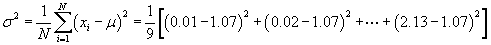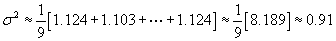Now, we can calculate the standard deviation, σ.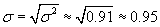Sample Variance and Standard Deviation

The formulas above are for populations (a concept we discussed in the previous article). But what about for samples? When we use a sample rather than a full population, we are considering only a portion of all the potentially available data. As a result, our statistical description of the sample may not align exactly with the population at large. Hence, we must take this into consideration when calculating the sample variance and sample standard deviation. Formulas (and the resulting values) that attempt to calculate a statistical value for a population on the basis of a sample of that population are called estimators.

As it turns out, one generally accepted method of accounting for this potential discrepancy is to adjust the coefficient in front of the summation. By subtracting unity from the number of data values n in the sample, we are effectively increasing the variance (and standard deviation) slightly. This adjustment attempts to account for values in the population that are not included in the sample. For sample statistics, we use Roman letters rather than Greek characters; thus, the sample variance is s2, and the sample standard deviation is s. (We represent the mean as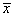.)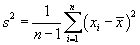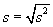Practice Problem: A statistician is considering the ages (in years) of a group of people chosen at random from a large city. The data she collected are shown below; on the basis of these data, what is the variance of the ages of people in the city?

{50, 24, 13, 47, 62, 81, 56, 35, 39, 28}

Solution: Clearly, the data above do not correspond to the entire population of a large city. Assuming that the data are representative, however, we can use an estimator of the population variance-specifically, we'll use the sample variance. First, we must calculate the mean of the data set.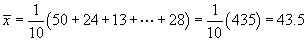The mean age is thus 43.5 years. Next, we can use our formula for the sample variance to calculate the answer to the problem.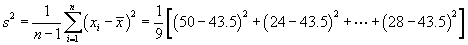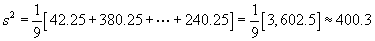Note that the units of this result are squared years. The standard deviation (in years) would be 20.

Skewness (Asymmetry)

In addition to measures of dispersion, we may also wish to characterize the asymmetry of a data distribution about the mean. Skewness is one such measure of asymmetry, and we can use the third moment about the mean to help represent it mathematically. We use the symbol γ to represent skewness.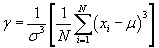Notice that the term in brackets is the third moment about the mean. This term is divided by σ3, which is the third power of the standard deviation. In a sense, this division "normalizes" the third moment. If the data are distributed about the mean in a symmetrical manner, the skewness of the data set is zero. A negative skewness indicates that the data set is distributed such that it is skewed toward values higher than the mean, whereas a positive skewness indicates the opposite. (Another way of looking at skewness in this case is that a negative skewness means that data values less than the mean tend to be farther away from the mean than data values greater than the mean. Positive skewness involves the opposite.) Skewness is best illustrated by way of histograms or similar representations-this is a topic that requires an understanding of frequencies, however. For the purposes of this article, we will only consider the population skewness.

Practice Problem: Calculate the skewness of the following data set (assume the data set corresponds to a population).

{1, 3, 5, 6, 6, 8, 10, 15}

Solution: To calculate the skewness, we must first find both the mean and the standard deviation. Let's start with the mean: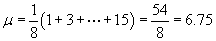Next, we'll calculate the variance and standard deviation.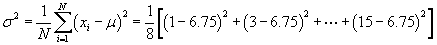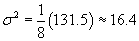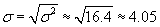Now, let's calculate the skewness, γ.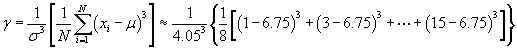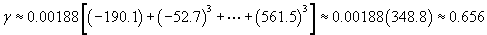Note that this means that the data set is "skewed" toward the values less than the mean.